MathScore EduFighter is one of the best math games on the Internet today. You can start playing for free!

## Fraction Comparison - Sample Math Practice Problems

The math problems below can be generated by MathScore.com, a math practice program for schools and individual families. References to complexity and mode refer to the overall difficulty of the problems as they appear in the main program. In the main program, all problems are automatically graded and the difficulty adapts dynamically based on performance. Answers to these sample questions appear at the bottom of the page. This page does not grade your responses.

See some of our other supported math practice problems.

### Complexity=2, Mode=same-v

Use >, <, or = to relate the two fractions.

1.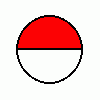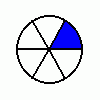12
 16
2.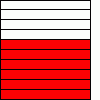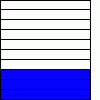610
 310

### Complexity=2, Mode=bench-v

Use >, <, or = to relate the two fractions.

1.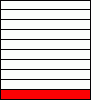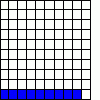110
 9100
2.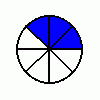12
 38

### Complexity=2, Mode=-v

Use >, <, or = to relate the two fractions.

1.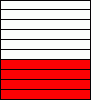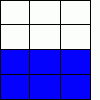410
 612
2.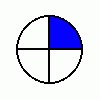12
 14

### Complexity=2

Use >, <, or = to relate the two fractions.

1.
 210
 12
2.
 12
 45

### Complexity=2, Mode=same-v

Use >, <, or = to relate the two fractions.

112
 16
Solution
When the numerators are the same, compare the denominators.
The smaller the number in the denominator, the larger each fractional part is. (see diagram)
This is because the whole is divided into fewer parts so each part is larger.
So,
 12
>
 16
2610
 310
Solution
When the denominators are the same, compare the numerators.
6 > 3
So,
 610
>
 310

### Complexity=2, Mode=bench-v

Use >, <, or = to relate the two fractions.

1110
 9100
Solution
When comparing fractions with different denominators, find equivalent fractions with like denominators to compare.
Find a common denominator:
For 10:   10 × 10 = 100.
For 100:   100 × 1 = 100.

Find equivalent fractions:
 110
× 10
× 10
=
 10100
 9100
Compare:
 10100
>
 9100
212
 38
Solution
When comparing fractions with different denominators, find equivalent fractions with like denominators to compare.
Find a common denominator:
For 2:   2 × 4 = 8.
For 8:   8 × 1 = 8.

Find equivalent fractions:
 12
× 4
× 4
=
 48
 38
Compare:
 48
>
 38

### Complexity=2, Mode=-v

Use >, <, or = to relate the two fractions.

1410
 612
Solution
Simplify 4/10 to 2/5.
Simplify 6/12 to 1/2.
So you are now comparing 2/5 and 1/2
When comparing fractions with different denominators, find equivalent fractions with like denominators to compare.
Find a common denominator:
For 5:   5 × 2 = 10.
For 2:   2 × 5 = 10.

Find equivalent fractions:
 25
× 2
× 2
=
 410
 12
× 5
× 5
=
 510
Compare:
 410
<
 510
212
 14
Solution
When the numerators are the same, compare the denominators.
The smaller the number in the denominator, the larger each fractional part is. (see diagram)
This is because the whole is divided into fewer parts so each part is larger.
So,
 12
>
 14

### Complexity=2

Use >, <, or = to relate the two fractions.

1
 210
 12
Solution
When comparing fractions with different denominators, find equivalent fractions with like denominators to compare.
Find a common denominator:
For 10:   10 × 1 = 10.
For 2:   2 × 5 = 10.

Find equivalent fractions:
 210
 12
× 5
× 5
=
 510
Compare:
 210
<
 510
2
 12
 45
Solution
When comparing fractions with different denominators, find equivalent fractions with like denominators to compare.
Find a common denominator:
For 2:   2 × 5 = 10.
For 5:   5 × 2 = 10.

Find equivalent fractions:
 12
× 5
× 5
=
 510
 45
× 2
× 2
=
 810
Compare:
 510
<
 810